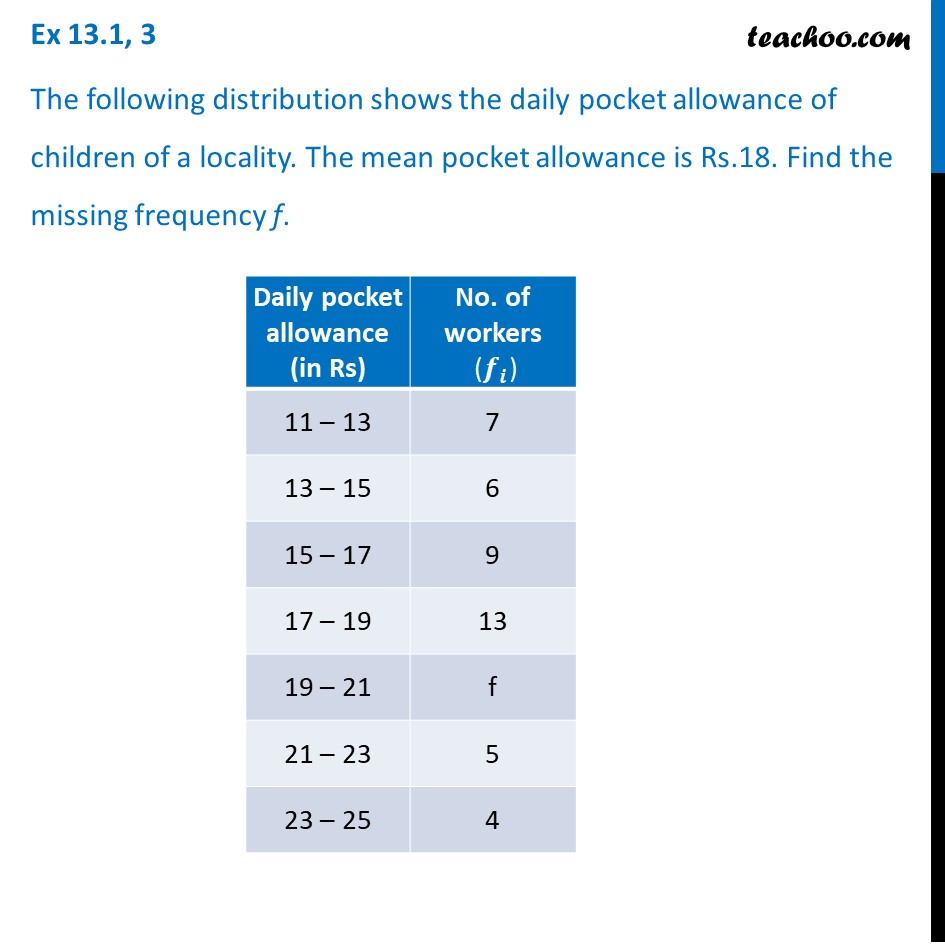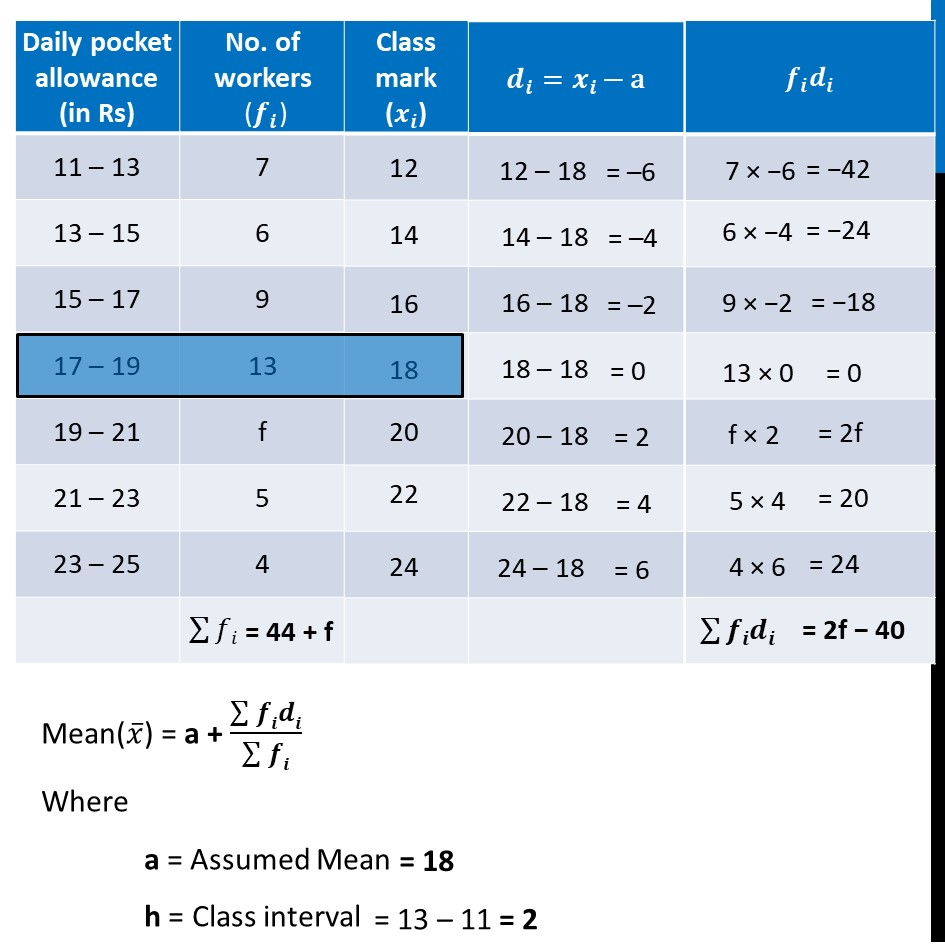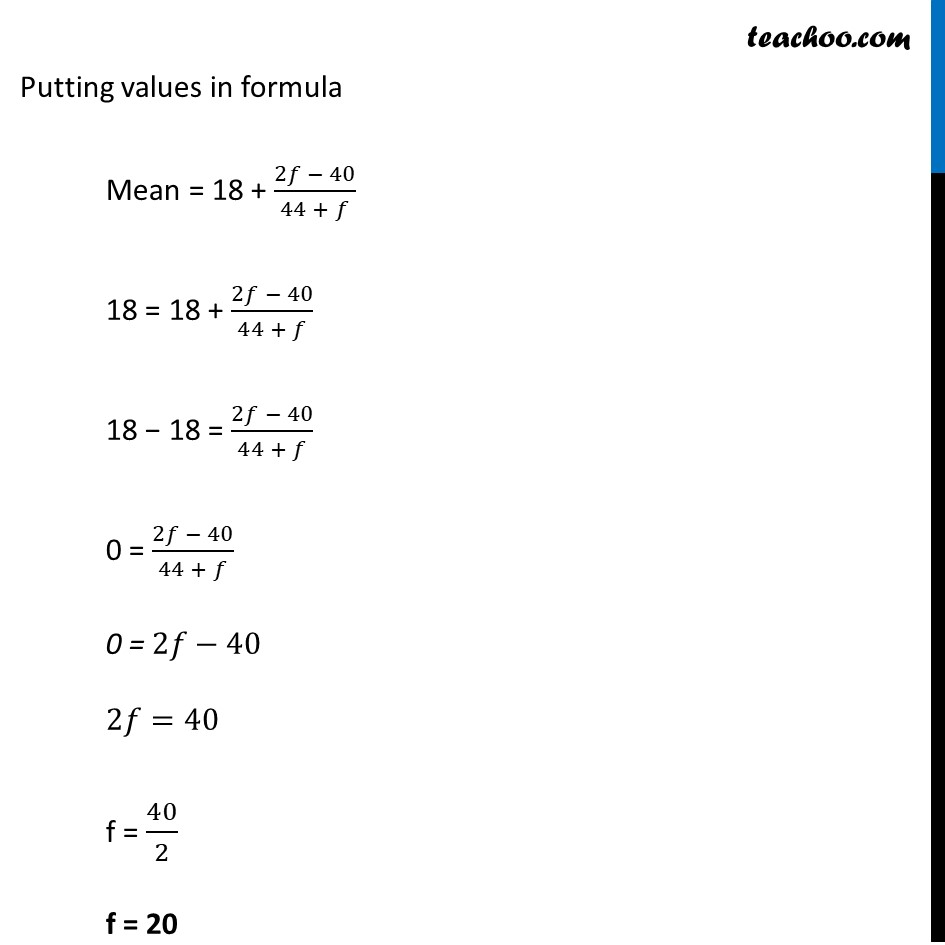Chapter 14 Class 10 Statistics

Class 10
Important Questions for Exam - Class 10Learn in your speed, with individual attention - Teachoo Maths 1-on-1 Class

### Transcript

Ex 13.1, 3 The following distribution shows the daily pocket allowance of children of a locality. The mean pocket allowance is Rs.18. Find the missing frequency f. Mean(𝑥 ̅) = a + (∑▒𝒇𝒊𝒅𝒊)/(∑▒𝒇𝒊) Where a = Assumed Mean h = Class interval Putting values in formula Mean = 18 + (2𝑓 − 40)/(44 + 𝑓) 18 = 18 + (2𝑓 − 40)/(44 + 𝑓) 18 − 18 = (2𝑓 − 40)/(44 + 𝑓) 0 = (2𝑓 − 40)/(44 + 𝑓) 0 = 2𝑓−40 2𝑓=40 f = 40/2 f = 20